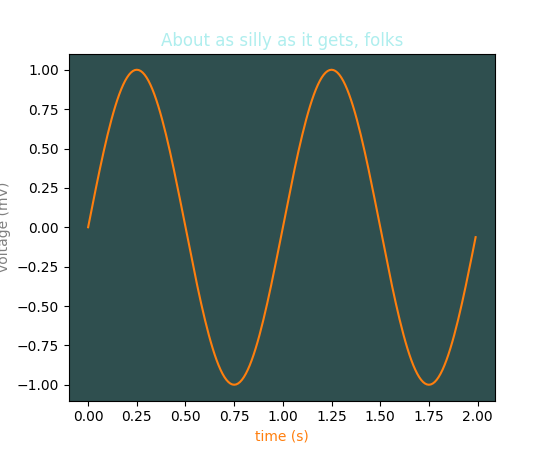Travis-CI:# pylab_examples example code: color_demo.py¶```"""
matplotlib gives you 5 ways to specify colors,

1) as a single letter string, ala MATLAB

2) as an html style hex string or html color name

3) as an R,G,B tuple, where R,G,B, range from 0-1

4) as a string representing a floating point number
from 0 to 1, corresponding to shades of gray.

5) as a special color "Cn", where n is a number 0-9 specifying the
nth color in the currently active color cycle.

"""
import matplotlib.pyplot as plt
import numpy as np

plt.subplot(111, facecolor='darkslategray')
#subplot(111, facecolor='#ababab')
t = np.arange(0.0, 2.0, 0.01)
s = np.sin(2*np.pi*t)
plt.plot(t, s, 'C1')
plt.xlabel('time (s)', color='C1')
plt.ylabel('voltage (mV)', color='0.5')  # grayscale color
plt.title('About as silly as it gets, folks', color='#afeeee')
plt.show()
```

Keywords: python, matplotlib, pylab, example, codex (see Search examples)